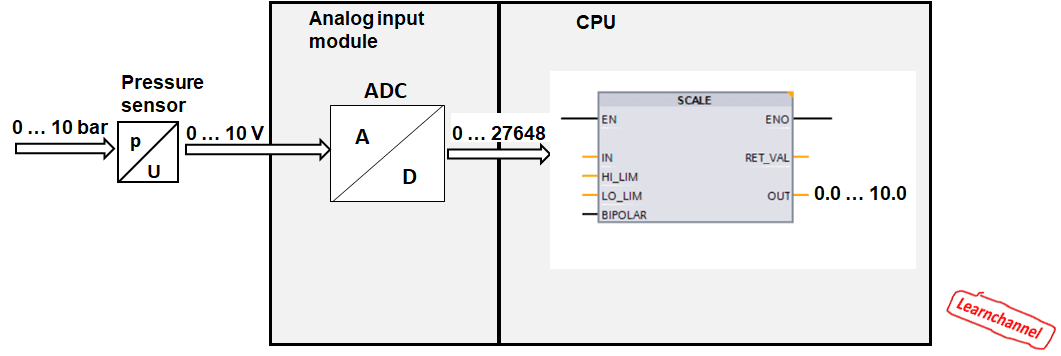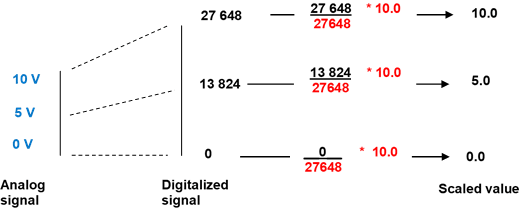# PLC – Scaling Analog values

### PLC - Normalizing, scaling analog values

The scanned range of 0-10V voltage or 0-20 mA current for analog modules from Siemens (SM 334) values ​​are illustrated in the following. Also can be seen, that 10 V / 20 mA digitalized has the valor of 2764810.PLC - Normalizing, scaling analog values

These digitized values ​​can be more easily processed in the PLC when they are normalized / scaled. Normalizing (scaling) means converting the integer value (from the analog input 'PIW'), into a real number, which refers to the voltage on the analog input or analog output. For example, measuring a voltage of 0-10 V should correspond to the actual number from 0.0 to 10.0. The algorithm for this conversion is quite simple:PLC - Scaling analog values

Work order:

Write a program that implements this algorithm.